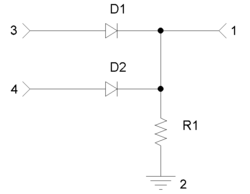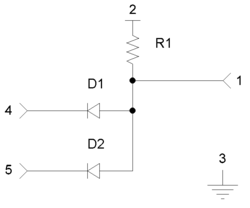# Diode logic

﻿
Diode logic

Diode logic (DL) or diode-resistor logic constructs Boolean logic gates from diodes acting as electrically operated switches. While diode logic has the advantage of simplicity, the lack of an amplifying stage in each gate limits its application. Not all logical functions can be implemented in diode logic alone; only the non-inverting logical AND and logical OR functions can be realized by diode gates. If several diode logic gates are cascaded, the voltage levels at each stage are significantly changed, so one-stage applications are used.

## Diode logic gate versions

In logic gates, logical functions are performed by parallel or series connected switches controlled by input logical variables. In diode logic, electrically operated switches are implemented by diodes: when forward biased, a diode switch is closed; when backward biased, the switch is open. There are two kinds of diode logic gates - OR and AND. It is not possible to construct NOT diode gate. The explanations below are true for positive logic (high voltage represents logical 1 and low voltage represents logical 0).

### OR logic gateIn a diode OR gate, the output voltage is high if at least one input voltage is high. The output voltage is low if all the input voltages are low.

OR logic gates are implemented by parallel connected normally open switches. So, in diode OR logic gates, the input voltage sources are connected to diode anodes. Diode cathodes are joined to the output (node 1 in the figure), which is connected through the pull-down resistor R1 to ground.

Input logical one. If the voltage of a particular input voltage source is high (input logical 1), the according diode is forward biased and this diode switch is closed. The input source passes current through the diode and creates high voltage drop across the resistor R1 (output logical 1). The rest of diodes connected to low input voltage (input logical 0s) are backward biased and their input sources (grounds) are disconnected from the output.

Input logical zeros. If all the input voltages are low (input logical 0), the voltage drops across the diodes are zero. These diode switches are open and the input sources (grounds) are disconnected from the output. No current flows through the resistor. The output voltage is low (output logical 0) and the output resistance is R1.

If two diode OR logic gates are cascaded, they behave as current-sourcing logic gates: if the first gate produces high output voltage, the second gate consumes current from the first one. If the first gate produces low output voltage, the second gate does not inject current into the output of the first one. A diode OR gate does not use its own power supply. The input sources with high voltage (logical 1) supply the load through the forward-biased diodes.

### AND logic gate

#### Basic idea

AND logic gates are implemented by series connected normally open switches. So, diode AND logic gates should be implemented by series connected diode switches (like an NMOS AND gate that is implemented by series connected transistor switches). However, in contrast to transistors, diodes are two-terminal switching elements, in which the input and output are not separated but they are the same. As a result, series connected diode switches cannot be driven by grounded input voltage sources. To solve this problem, diode AND gates are constructed in the same manner as OR diode gates - by parallel connected diode switches.[nb 1] However, to obtain AND instead OR function according to De Morgan's laws, the input and output logical variables are inverted:

Y = NOT (NOT (X1) OR NOT (X2)) = NOT (NOT (X1 AND X2)) = X1 AND X2,

where X1 and X2 are the two input logical variables; Y is the output variable.

Therefore, the diode AND logic gate is a modified diode OR logic gate: the diode AND gate is actually a diode OR gate with inverted inputs and output.

#### ImplementationIn a diode AND gate, the output voltage is high if all the input voltages are high. The output voltage is low if at least one of the input voltage is low.

To realize the basic idea, the diodes are reverse connected and forward biased by an additional voltage source +V (a power supply) through the pull-up resistor R1. The input voltage sources are connected in opposite direction to the supplying voltage source (traveling along the loop +V - R1 - D - Vin). To invert the output voltage and to get a grounded output, the complementary voltage drop (+V - VR1) between the output and ground is taken as an output instead the floating voltage drop VR1 across the resistor.

Input logical ones. When all the input voltages are high, they "neutralize" the biasing supply voltage +V. The voltage drops across the diodes are zero and these diode switches are open. The output voltage is high (output logical 1) since no current flows through the resistor and there is no voltage drop across it. The output resistance is R1. Hence, the behavior of the diode switches is reversed - whereas in diode OR logic gates diodes act as normally open switches, in diode AND logic gates diodes act as normally closed switches.

Input logical zero. If the voltage of some input voltage source is low (input logical 0), the power supply passes current through the resistor, diode and the input source. The diode is forward biased (the diode switch is closed) and the output voltage drop across the diode is low (output logical 0). The output resistance is low and is determined by the input source. The rest of diodes connected to high input voltages (input logical 1s) are backward biased and their input sources are disconnected from the output Node 1.

If two diode AND logic gates are cascaded, they behave as current-sinking logic gates: if the first gate produces high output voltage, the second gate does not consume current from the first one; if the first gate produces low output voltage, the second gate injects current into the output of the first one. A diode AND gate uses its own power supply to drive the load through the pull-up resistor.

## Properties

### Non-restoring logicIn cascaded AND-OR diode gates, the high voltage level is decreased more than two times.

Digital logic implemented by active elements is characterized by signal restoration. True and false or 1 and 0 are represented by two specific voltage levels. If the inputs to a digital logic gate is close to their respective levels, the output will be closer or exactly equal to its desired level. Active logic gates may be integrated in large numbers because each gate tends to remove noise at its input. Diode logic gates are implemented by passive elements; so, they have two restoration problems.

Forward voltage drop. The first restoration problem of diode logic is that there is a voltage drop VF about 0.6 V across the forward-biased diode. This voltage is added to or subtracted from the input of every gate so that it accumulates when identical diode gates are cascaded. In an OR gate, VF decreases the high voltage level (the logical 1) while in an AND gate, it increases the low voltage level (the logical 0). The feasible number of logic stages thus depends on the difference between the high and low voltages.

Source resistance. Another problem of diode logic is the internal resistance of the input voltage sources. Together with the gate resistor, it constitutes a voltage divider that worsens the voltage levels. In an OR gate, the source resistance decreases the high voltage level (the logical 1) while in an AND gate, it increases the low voltage level (the logical 0). In the cascaded AND-OR diode gates in the picture on the right, the AND high output voltages are decreased because of the internal voltage drops across the AND pull-up resistances.

### Non-inverting logic

Diode logic is non-inverting in both the OR and AND configurations: a diode OR gate is true non-inverting (Y = X in the case of one-input OR gate) while a diode AND gate is non-inverting since it is double inverting (Y = NOT ((NOT (X)) = X in the case of one-input AND gate - see the considerations above). Diode AND gate would be inverting if the voltage drop across the resistor is taken as an output but the load would be not grounded in this case.

## Applications

Diode logic gates were used to build diode-transistor logic gates (DTL) as integrated circuits.

The outputs of conventional ICs (with complementary output stages) must never be directly connected together since they act as voltage sources.[nb 2] However, diodes can be used to combine two or more digital (high/low) outputs from an IC such as a counter. This wired logic connection can be a useful way of producing simple logic functions without using additional logic gates.

Wikimedia Foundation. 2010.

### Look at other dictionaries:

• transistor-diode logic — loginis diodinis tranzistorinis grandynas statusas T sritis radioelektronika atitikmenys: angl. diode transistor logic; transistor diode logic vok. Dioden Transistor Logik, f; Transistor Dioden Logik, f rus. диодно транзисторная логика, f; диодно …   Radioelektronikos terminų žodynas

• Logic (disambiguation) — Logic is the study of the principles and criteria of valid inference and demonstration.Logic may also refer to:In logic and mathematics*A branch of logic: **Inductive logic, also called induction or inductive reasoning **Informal logic, the study …   Wikipedia

• Logic family — In computer engineering, a logic family may refer to one of two related concepts. A logic family of monolithic digital integrated circuit devices is a group of electronic logic gates constructed using one of several different designs, usually… …   Wikipedia

• Diode–transistor logic — DTL redirects here. For other uses, see DTL (disambiguation). Diode–transistor logic (DTL) is a class of digital circuits that is the direct ancestor of transistor–transistor logic. It is called so because the logic gating function (e.g., AND) is …   Wikipedia

• Logic gate — A logic gate is an idealized or physical device implementing a Boolean function, that is, it performs a logical operation on one or more logic inputs and produces a single logic output. Depending on the context, the term may refer to an ideal… …   Wikipedia

• Diode — Figure 1: Closeup of a diode, showing the square shaped semiconductor crystal (black object on left) …   Wikipedia

• diode-transistor logic — loginis diodinis tranzistorinis grandynas statusas T sritis radioelektronika atitikmenys: angl. diode transistor logic; transistor diode logic vok. Dioden Transistor Logik, f; Transistor Dioden Logik, f rus. диодно транзисторная логика, f; диодно …   Radioelektronikos terminų žodynas

• Diode-or circuit — A diode OR circuit is used in electronics to isolate two or more voltage sources. There are two typical implementations: When a DC supply voltage needs to be generated from one of a number of different sources, for example when terminating a… …   Wikipedia

• Diode matrix — A diode matrix is a two dimensional grid of wires, where each intersection where one row crosses over another either has a diode connecting them, or the wires are isolated from each other. It is one of the most popular techniques for implementing …   Wikipedia

• diode-transistor logic with Zener diodes — loginis diodinis tranzistorinis grandynas su Zenerio diodais statusas T sritis radioelektronika atitikmenys: angl. diode transistor logic with Zener diodes vok. Dioden Transistor Logik mit Zener Dioden, f rus. диодно транзисторная логическая… …   Radioelektronikos terminų žodynas